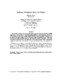## Stabbing Orthogonal Objects in 3-Space##### Files
CS-TR-3701.ps(652.48 KB)
We consider a problem that arises in the design of data structures for answering {\em visibility range queries}, that is, given a $3$-dimensional scene defined by a set of polygonal patches, we wish to preprocess the scene to answer queries involving the set of patches of the scene that are visible from a given range of points over a given range of viewing directions. These data structures recursively subdivide space into cells until some criterion is satisfied. One of the important problems that arise in the construction of such data structures is that of determining whether a cell represents a nonempty region of space, and more generally computing the size of a cell. In this paper we introduce a measure of the {\em size} of the subset of lines in 3-space that stab a given set of $n$ polygonal patches, based on the maximum angle and distance between any two lines in the set. Although the best known algorithm for computing this size measure runs in $O(n^2)$ time, we show that if the polygonal patches are orthogonal rectangles, then this measure can be approximated to within a constant factor in $O(n)$ time. (Also cross-referenced as UMIACS-TR-96-71)